Elementary Algebra 2e

# 7.4Factor Special Products

Elementary Algebra 2e7.4 Factor Special Products

### Learning Objectives

By the end of this section, you will be able to:

• Factor perfect square trinomials
• Factor differences of squares
• Factor sums and differences of cubes
• Choose method to factor a polynomial completely

### Be Prepared 7.11

Before you get started, take this readiness quiz.

Simplify: $(12x)2.(12x)2.$
If you missed this problem, review Example 6.23.

### Be Prepared 7.12

Multiply: $(m+4)2.(m+4)2.$
If you missed this problem, review Example 6.47.

### Be Prepared 7.13

Multiply: $(p−9)2.(p−9)2.$
If you missed this problem, review Example 6.48.

### Be Prepared 7.14

Multiply: $(k+3)(k−3).(k+3)(k−3).$
If you missed this problem, review Example 6.52.

The strategy for factoring we developed in the last section will guide you as you factor most binomials, trinomials, and polynomials with more than three terms. We have seen that some binomials and trinomials result from special products—squaring binomials and multiplying conjugates. If you learn to recognize these kinds of polynomials, you can use the special products patterns to factor them much more quickly.

### Factor Perfect Square Trinomials

Some trinomials are perfect squares. They result from multiplying a binomial times itself. You can square a binomial by using FOIL, but using the Binomial Squares pattern you saw in a previous chapter saves you a step. Let’s review the Binomial Squares pattern by squaring a binomial using FOIL.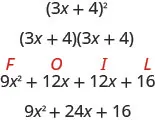The first term is the square of the first term of the binomial and the last term is the square of the last. The middle term is twice the product of the two terms of the binomial.

$(3x)2+2(3x·4)+429x2+24x+16(3x)2+2(3x·4)+429x2+24x+16$

The trinomial 9x2 + 24 +16 is called a perfect square trinomial. It is the square of the binomial 3x+4.

We’ll repeat the Binomial Squares Pattern here to use as a reference in factoring.

### Binomial Squares Pattern

If a and b are real numbers,

$(a+b)2=a2+2ab+b2(a−b)2=a2−2ab+b2(a+b)2=a2+2ab+b2(a−b)2=a2−2ab+b2$

When you square a binomial, the product is a perfect square trinomial. In this chapter, you are learning to factor—now, you will start with a perfect square trinomial and factor it into its prime factors.

You could factor this trinomial using the methods described in the last section, since it is of the form ax2 + bx + c. But if you recognize that the first and last terms are squares and the trinomial fits the perfect square trinomials pattern, you will save yourself a lot of work.

Here is the pattern—the reverse of the binomial squares pattern.

### Perfect Square Trinomials Pattern

If a and b are real numbers,

$a2+2ab+b2=(a+b)2a2−2ab+b2=(a−b)2a2+2ab+b2=(a+b)2a2−2ab+b2=(a−b)2$

To make use of this pattern, you have to recognize that a given trinomial fits it. Check first to see if the leading coefficient is a perfect square, $a2a2$. Next check that the last term is a perfect square, $b2b2$. Then check the middle term—is it twice the product, 2ab? If everything checks, you can easily write the factors.

### Example 7.42

#### How to Factor Perfect Square Trinomials

Factor: $9x2+12x+49x2+12x+4$.

### Try It 7.83

Factor: $4x2+12x+94x2+12x+9$.

### Try It 7.84

Factor: $9y2+24y+169y2+24y+16$.

The sign of the middle term determines which pattern we will use. When the middle term is negative, we use the pattern $a2−2ab+b2a2−2ab+b2$, which factors to $(a−b)2(a−b)2$.

The steps are summarized here.

### How To

#### Factor perfect square trinomials.

$Step 1.Does the trinomial fit the pattern?a2+2ab+b2a2−2ab+b2•Is the first term a perfect square?(a)2(a)2Write it as a square.•Is the last term a perfect square?(a)2(b)2(a)2(b)2Write it as a square.•Check the middle term. Is it2ab? (a)2↘2·a·b↙(b)2(a)2↘2·a·b↙(b)2 Step 2.Write the square of the binomial.(a+b)2(a−b)2Step 3.Check by multiplying.Step 1.Does the trinomial fit the pattern?a2+2ab+b2a2−2ab+b2•Is the first term a perfect square?(a)2(a)2Write it as a square.•Is the last term a perfect square?(a)2(b)2(a)2(b)2Write it as a square.•Check the middle term. Is it2ab? (a)2↘2·a·b↙(b)2(a)2↘2·a·b↙(b)2 Step 2.Write the square of the binomial.(a+b)2(a−b)2Step 3.Check by multiplying.$

We’ll work one now where the middle term is negative.

### Example 7.43

Factor: $81y2−72y+1681y2−72y+16$.

### Try It 7.85

Factor: $64y2−80y+2564y2−80y+25$.

### Try It 7.86

Factor: $16z2−72z+8116z2−72z+81$.

The next example will be a perfect square trinomial with two variables.

### Example 7.44

Factor: $36x2+84xy+49y236x2+84xy+49y2$.

### Try It 7.87

Factor: $49x2+84xy+36y249x2+84xy+36y2$.

### Try It 7.88

Factor: $64m2+112mn+49n264m2+112mn+49n2$.

### Example 7.45

Factor: $9x2+50x+259x2+50x+25$.

### Try It 7.89

Factor: $16r2+30rs+9s216r2+30rs+9s2$.

### Try It 7.90

Factor: $9u2+87u+1009u2+87u+100$.

Remember the very first step in our Strategy for Factoring Polynomials? It was to ask “is there a greatest common factor?” and, if there was, you factor the GCF before going any further. Perfect square trinomials may have a GCF in all three terms and it should be factored out first. And, sometimes, once the GCF has been factored, you will recognize a perfect square trinomial.

### Example 7.46

Factor: $36x2y−48xy+16y36x2y−48xy+16y$.

### Try It 7.91

Factor: $8x2y−24xy+18y8x2y−24xy+18y$.

### Try It 7.92

Factor: $27p2q+90pq+75q27p2q+90pq+75q$.

### Factor Differences of Squares

The other special product you saw in the previous chapter was the Product of Conjugates pattern. You used this to multiply two binomials that were conjugates. Here’s an example:

$(3x−4)(3x+4)9x2−16(3x−4)(3x+4)9x2−16$

Remember, when you multiply conjugate binomials, the middle terms of the product add to 0. All you have left is a binomial, the difference of squares.

Multiplying conjugates is the only way to get a binomial from the product of two binomials.

### Product of Conjugates Pattern

If a and b are real numbers

$(a−b)(a+b)=a2−b2(a−b)(a+b)=a2−b2$

The product is called a difference of squares.

To factor, we will use the product pattern “in reverse” to factor the difference of squares. A difference of squares factors to a product of conjugates.

### Difference of Squares Pattern

If a and b are real numbers,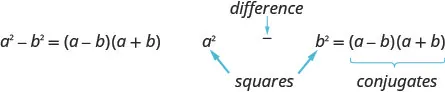Remember, “difference” refers to subtraction. So, to use this pattern you must make sure you have a binomial in which two squares are being subtracted.

### Example 7.47

#### How to Factor Differences of Squares

Factor: $x2−4x2−4$.

### Try It 7.93

Factor: $h2−81h2−81$.

### Try It 7.94

Factor: $k2−121k2−121$.

### How To

#### Factor differences of squares.

$Step 1.Does the binomial fit the pattern?a2−b2•Is this a difference?____−____•Are the first and last terms perfect squares?Step 2.Write them as squares.(a)2−(b)2Step 3.Write the product of conjugates.(a−b)(a+b)Step 4.Check by multiplying.Step 1.Does the binomial fit the pattern?a2−b2•Is this a difference?____−____•Are the first and last terms perfect squares?Step 2.Write them as squares.(a)2−(b)2Step 3.Write the product of conjugates.(a−b)(a+b)Step 4.Check by multiplying.$

It is important to remember that sums of squares do not factor into a product of binomials. There are no binomial factors that multiply together to get a sum of squares. After removing any GCF, the expression $a2+b2a2+b2$ is prime!

Don’t forget that 1 is a perfect square. We’ll need to use that fact in the next example.

### Example 7.48

Factor: $64y2−164y2−1$.

### Try It 7.95

Factor: $m2−1m2−1$.

### Try It 7.96

Factor: $81y2−181y2−1$.

### Example 7.49

Factor: $121x2−49y2121x2−49y2$.

### Try It 7.97

Factor: $196m2−25n2196m2−25n2$.

### Try It 7.98

Factor: $144p2−9q2144p2−9q2$.

The binomial in the next example may look “backwards,” but it’s still the difference of squares.

### Example 7.50

Factor: $100−h2100−h2$.

### Try It 7.99

Factor: $144−x2144−x2$.

### Try It 7.100

Factor: $169−p2169−p2$.

To completely factor the binomial in the next example, we’ll factor a difference of squares twice!

### Example 7.51

Factor: $x4−y4x4−y4$.

### Try It 7.101

Factor: $a4−b4a4−b4$.

### Try It 7.102

Factor: $x4−16x4−16$.

As always, you should look for a common factor first whenever you have an expression to factor. Sometimes a common factor may “disguise” the difference of squares and you won’t recognize the perfect squares until you factor the GCF.

### Example 7.52

Factor: $8x2y−98y8x2y−98y$.

### Try It 7.103

Factor: $7xy2−175x7xy2−175x$.

### Try It 7.104

Factor: $45a2b−80b45a2b−80b$.

### Example 7.53

Factor: $6x2+966x2+96$.

### Try It 7.105

Factor: $8a2+2008a2+200$.

### Try It 7.106

Factor: $36y2+8136y2+81$.

### Factor Sums and Differences of Cubes

There is another special pattern for factoring, one that we did not use when we multiplied polynomials. This is the pattern for the sum and difference of cubes. We will write these formulas first and then check them by multiplication.

$a3+b3=(a+b)(a2−ab+b2)a3−b3=(a−b)(a2+ab+b2)a3+b3=(a+b)(a2−ab+b2)a3−b3=(a−b)(a2+ab+b2)$

We’ll check the first pattern and leave the second to you.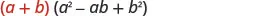Distribute.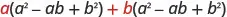Multiply. $a3−a2b+ab2+a2b−ab2+b3a3−a2b+ab2+a2b−ab2+b3$ Combine like terms. $a3+b3a3+b3$

### Sum and Difference of Cubes Pattern

$a3+b3=(a+b)(a2−ab+b2)a3−b3=(a−b)(a2+ab+b2)a3+b3=(a+b)(a2−ab+b2)a3−b3=(a−b)(a2+ab+b2)$

The two patterns look very similar, don’t they? But notice the signs in the factors. The sign of the binomial factor matches the sign in the original binomial. And the sign of the middle term of the trinomial factor is the opposite of the sign in the original binomial. If you recognize the pattern of the signs, it may help you memorize the patterns.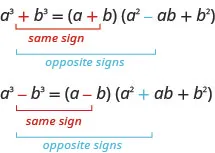The trinomial factor in the sum and difference of cubes pattern cannot be factored.

It can be very helpful if you learn to recognize the cubes of the integers from 1 to 10, just like you have learned to recognize squares. We have listed the cubes of the integers from 1 to 10 in Table 7.2.

n 1 2 3 4 5 6 7 8 9 10
$n3n3$ 1 8 27 64 125 216 343 512 729 1000
Table 7.2

### Example 7.54

#### How to Factor the Sum or Difference of Cubes

Factor: $x3+64x3+64$.

### Try It 7.107

Factor: $x3+27x3+27$.

### Try It 7.108

Factor: $y3+8y3+8$.

### How To

#### Factor the sum or difference of cubes.

To factor the sum or difference of cubes:

1. Step 1.
Does the binomial fit the sum or difference of cubes pattern?
• Is it a sum or difference?
• Are the first and last terms perfect cubes?
2. Step 2. Write them as cubes.
3. Step 3. Use either the sum or difference of cubes pattern.
4. Step 4. Simplify inside the parentheses
5. Step 5. Check by multiplying the factors.

### Example 7.55

Factor: $x3−1000x3−1000$.

### Try It 7.109

Factor: $u3−125u3−125$.

### Try It 7.110

Factor: $v3−343v3−343$.

Be careful to use the correct signs in the factors of the sum and difference of cubes.

### Example 7.56

Factor: $512−125p3512−125p3$.

### Try It 7.111

Factor: $64−27x364−27x3$.

### Try It 7.112

Factor: $27−8y327−8y3$.

### Example 7.57

Factor: $27u3−125v327u3−125v3$.

### Try It 7.113

Factor: $8x3−27y38x3−27y3$.

### Try It 7.114

Factor: $1000m3−125n31000m3−125n3$.

In the next example, we first factor out the GCF. Then we can recognize the sum of cubes.

### Example 7.58

Factor: $5m3+40n35m3+40n3$.

### Try It 7.115

Factor: $500p3+4q3500p3+4q3$.

### Try It 7.116

Factor: $432c3+686d3432c3+686d3$.

### Media

Access these online resources for additional instruction and practice with factoring special products.

### Section 7.4 Exercises

#### Practice Makes Perfect

Factor Perfect Square Trinomials

In the following exercises, factor.

215.

$16 y 2 + 24 y + 9 16 y 2 + 24 y + 9$

216.

$25 v 2 + 20 v + 4 25 v 2 + 20 v + 4$

217.

$36 s 2 + 84 s + 49 36 s 2 + 84 s + 49$

218.

$49 s 2 + 154 s + 121 49 s 2 + 154 s + 121$

219.

$100 x 2 − 20 x + 1 100 x 2 − 20 x + 1$

220.

$64 z 2 − 16 z + 1 64 z 2 − 16 z + 1$

221.

$25 n 2 − 120 n + 144 25 n 2 − 120 n + 144$

222.

$4 p 2 − 52 p + 169 4 p 2 − 52 p + 169$

223.

$49 x 2 − 28 x y + 4 y 2 49 x 2 − 28 x y + 4 y 2$

224.

$25 r 2 − 60 r s + 36 s 2 25 r 2 − 60 r s + 36 s 2$

225.

$25 n 2 + 25 n + 4 25 n 2 + 25 n + 4$

226.

$100 y 2 − 20 y + 1 100 y 2 − 20 y + 1$

227.

$64 m 2 − 16 m + 1 64 m 2 − 16 m + 1$

228.

$100 x 2 − 25 x + 1 100 x 2 − 25 x + 1$

229.

$10 k 2 + 80 k + 160 10 k 2 + 80 k + 160$

230.

$64 x 2 − 96 x + 36 64 x 2 − 96 x + 36$

231.

$75 u 3 − 30 u 2 v + 3 u v 2 75 u 3 − 30 u 2 v + 3 u v 2$

232.

$90 p 3 + 300 p 2 q + 250 p q 2 90 p 3 + 300 p 2 q + 250 p q 2$

Factor Differences of Squares

In the following exercises, factor.

233.

$x 2 − 16 x 2 − 16$

234.

$n 2 − 9 n 2 − 9$

235.

$25 v 2 − 1 25 v 2 − 1$

236.

$169 q 2 − 1 169 q 2 − 1$

237.

$121 x 2 − 144 y 2 121 x 2 − 144 y 2$

238.

$49 x 2 − 81 y 2 49 x 2 − 81 y 2$

239.

$169 c 2 − 36 d 2 169 c 2 − 36 d 2$

240.

$36 p 2 − 49 q 2 36 p 2 − 49 q 2$

241.

$4 − 49 x 2 4 − 49 x 2$

242.

$121 − 25 s 2 121 − 25 s 2$

243.

$16 z 4 − 1 16 z 4 − 1$

244.

$m 4 − n 4 m 4 − n 4$

245.

$5 q 2 − 45 5 q 2 − 45$

246.

$98 r 3 − 72 r 98 r 3 − 72 r$

247.

$24 p 2 + 54 24 p 2 + 54$

248.

$20 b 2 + 140 20 b 2 + 140$

Factor Sums and Differences of Cubes

In the following exercises, factor.

249.

$x 3 + 125 x 3 + 125$

250.

$n 3 + 512 n 3 + 512$

251.

$z 3 − 27 z 3 − 27$

252.

$v 3 − 216 v 3 − 216$

253.

$8 − 343 t 3 8 − 343 t 3$

254.

$125 − 27 w 3 125 − 27 w 3$

255.

$8 y 3 − 125 z 3 8 y 3 − 125 z 3$

256.

$27 x 3 − 64 y 3 27 x 3 − 64 y 3$

257.

$7 k 3 + 56 7 k 3 + 56$

258.

$6 x 3 − 48 y 3 6 x 3 − 48 y 3$

259.

$2 − 16 y 3 2 − 16 y 3$

260.

$−2 x 3 − 16 y 3 −2 x 3 − 16 y 3$

Mixed Practice

In the following exercises, factor.

261.

$64 a 2 − 25 64 a 2 − 25$

262.

$121 x 2 − 144 121 x 2 − 144$

263.

$27 q 2 − 3 27 q 2 − 3$

264.

$4 p 2 − 100 4 p 2 − 100$

265.

$16 x 2 − 72 x + 81 16 x 2 − 72 x + 81$

266.

$36 y 2 + 12 y + 1 36 y 2 + 12 y + 1$

267.

$8 p 2 + 2 8 p 2 + 2$

268.

$81 x 2 + 169 81 x 2 + 169$

269.

$125 − 8 y 3 125 − 8 y 3$

270.

$27 u 3 + 1000 27 u 3 + 1000$

271.

$45 n 2 + 60 n + 20 45 n 2 + 60 n + 20$

272.

$48 q 3 − 24 q 2 + 3 q 48 q 3 − 24 q 2 + 3 q$

#### Everyday Math

273.

Landscaping Sue and Alan are planning to put a 15 foot square swimming pool in their backyard. They will surround the pool with a tiled deck, the same width on all sides. If the width of the deck is w, the total area of the pool and deck is given by the trinomial $4w2+60w+2254w2+60w+225$. Factor the trinomial.

274.

Home repair The height a twelve foot ladder can reach up the side of a building if the ladder’s base is b feet from the building is the square root of the binomial $144−b2144−b2$. Factor the binomial.

#### Writing Exercises

275.

Why was it important to practice using the binomial squares pattern in the chapter on multiplying polynomials?

276.

How do you recognize the binomial squares pattern?

277.

Explain why $n2+25≠(n+5)2n2+25≠(n+5)2$. Use algebra, words, or pictures.

278.

Maribel factored $y2−30y+81y2−30y+81$ as $(y−9)2(y−9)2$. Was she right or wrong? How do you know?

#### Self Check

After completing the exercises, use this checklist to evaluate your mastery of the objectives of this section.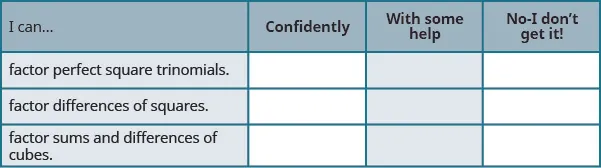On a scale of 1–10, how would you rate your mastery of this section in light of your responses on the checklist? How can you improve this?

Order a print copy

As an Amazon Associate we earn from qualifying purchases.